tabreturn.github.io

Processing.py in Ten Lessons – 04: Animation and Transformation

2018-08-10

Covered in this lesson:
Animation / Transformations / Time and Date / Animated Trigonometry

In this tutorial, you get to make things move. The content focuses primarily on animation, but also covers transformations, time & date functions, and some trigonometry. As you will discover, blending motion with math produces some exciting results.

Complete list of Processing.py lessons

Animation

Before writing any animation code, consider how motion is perceived. The brain is fed a snapshot from your retina around ten times each second. The speed at which objects appear to be moving (or not moving) is determined by the difference between successive snapshots. So, provided your screen can display a sequence of static images at a rate exceeding ten cycles per second, the viewer will experience the illusion of smooth flowing movement. This illusion is referred to as Beta movement and occurs at frame rates of around 10-12 images per second – although higher frame rates will appear even smoother. That said, there is more to motion perception than frames per second (fps).

Take a moment to note the numbering sequence of the circles in the illustration below.

Consider that you displayed just circle 1 for a full four seconds, followed by just circle 5 for another four seconds, looping the sequence indefinitely (an effective frame rate of 0.25 fps). The result, most observers would agree, is a pair of alternating images depicting circles in different positions. However, speed up the frame rate to around 2.5 fps, and one begins to interpret the sequence as a circle bouncing between two points. Speed up the frame rate further, and the two circles seemingly flicker in sync.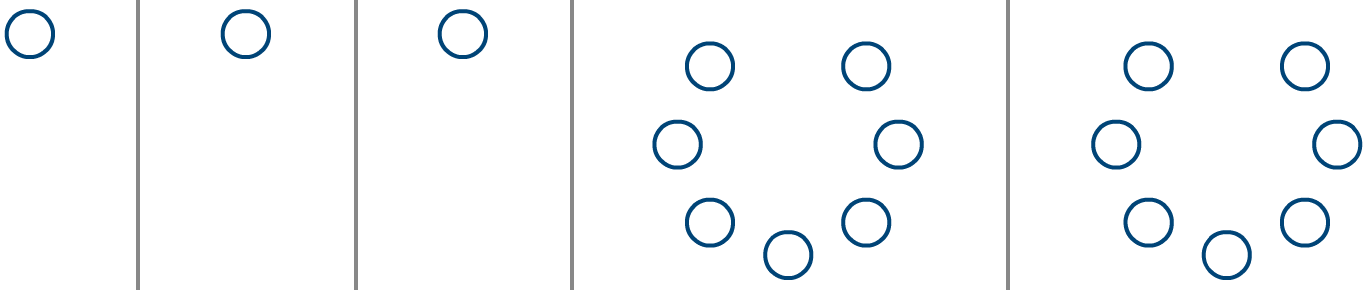Click the image to start the animation. Frame rates from left to right: 0.25 fps; 2.5 fps; 12 fps; 1 fps; 25 fps

The two rightmost animations (rings of circles) run at 1 and 25 fps. In the left/slower instance, the circle just ahead of a gap appears to jump into the void left by the vacant circle (if you didn’t see it this way before, you should now). In the more rapid animation, a phantom white dot appears to obscure the circles beneath it as it races around the ring – an illusion referred to as the phi phenomenon.

Animation Functions

All that is required to get animating in Processing are the setup() and draw() functions. Create a new sketch; save it as “beta_movement”; then add the following code:

The code resembles just about every other sketch you have set up thus far, excepting the def setup() line. Everything indented beneath the setup() is called once when the program starts. This area is used to define any initial properties, such as the display window size. Conversely, code indented beneath a draw() function is invoked with each new frame. Add a draw() function to see how this operates:

The frameCount is a system variable containing the number of frames displayed since starting the sketch. With each new frame, the draw() function calls the print function, which in-turn displays the current frame-count in the Console:

By default, the draw() executes at around 60 fps – but as the complexity of an animation increases, the frame rate is likely to drop. Adjust the frame rate using the frameRate() function (within the indented setup code), and add a condition to print on even frames only:

With the frameRate set to 2.5, the draw line runs two-and-a-half times every second; this means each frame is 400 milliseconds (0.4 of a second) in duration. Because the print line executes on every second frame, a new line appears in the Console every 800 milliseconds:

To draw a circle on every even frame, add an ellipse() line:

Now run the code. You may be surprised to find that the circle does not blink:

So why does the circle not disappear on odd frames? The reason is that everything in Processing persists after being drawn – so, every second frame another circle is drawn atop the existing ‘pile’. The background colour is defined within the setup() section, and is therefore drawn once; as it is drawn first, it is also the bottommost layer of this persistent arrangement. To ‘wipe’ each frame before drawing the next, simply move the background('#004477') line to your draw() section:

The result is a blinking circle. Using an else statement, add another circle with which this alternates:

You can now adjust the frame rate to test the effects.

For a more convincing effect, the animation should fill-in the intermediate frames.The onionskin rendition is used to indicate the frame positions.

To accomplish this without having to write out each frame position, one requires a variable to store and update the circle’s y value.

Global Variables

You will notice that the setup() and draw() functions are preceded by the def keyword. You will come to understand why this is in future lessons, but for now, need to be aware that variables cannot be directly shared between these two sections of code. Global variables address this challenge.

Create a new sketch and save it as “global_variables”. Add the following code:

Run the sketch and note the error message: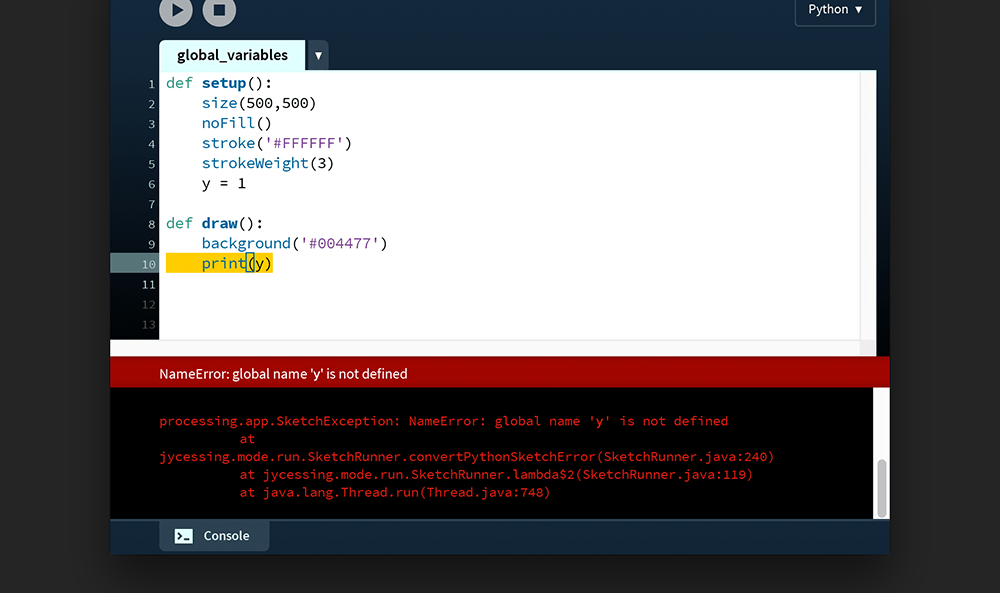"NameError: global name 'y' is not defined"

The y variable is defined within the setup() function. As such, y is only accessible among the indented lines of this block. The variable scope is therefore said to be local to setup(). Alternatively, one can place this variable line outside of the setup() function – thereby setting it in the global scope. This permits either function to read it. Stop writing code for a moment and consider the scenarios that follow.

Adding the y = 1 line to the top of the code places the variable in the global scope, making it accessible to the setup() and draw() functions:

However, this global y variable can be overridden on a local level by another variable named y. In the example below, the setup() function displays a 1, whereas the draw() function displays zeroes: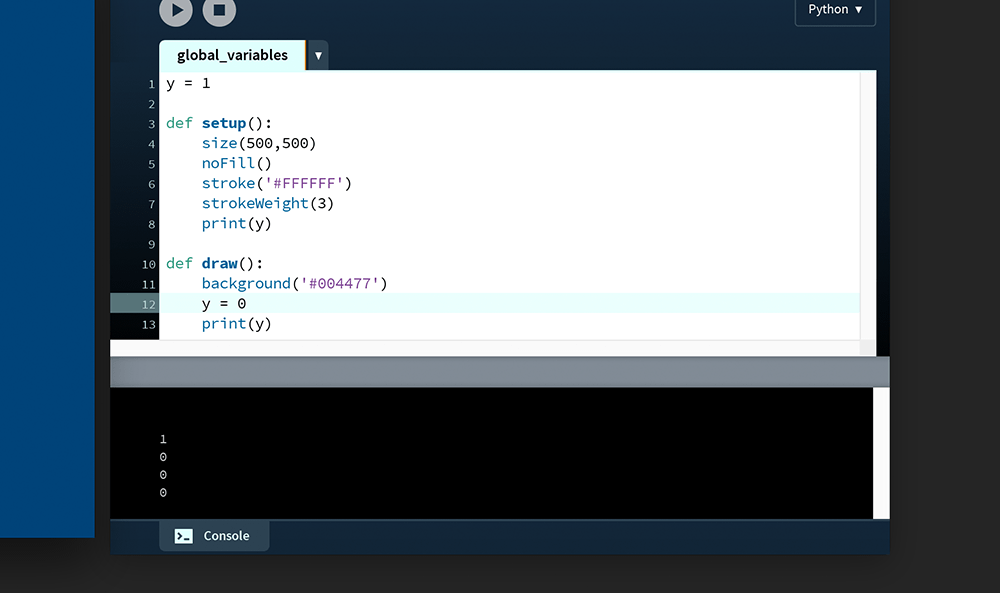The sketch has been run then stopped almost immediately. You will need to scroll to the very top line of the Console area to view the 1.

While you can override a global variable, you will find that you cannot write/reassign a value to it:

This is the point where the global keyword is useful. Edit your code, moving the y = 1 line to the top of your code (into the global scope). Then, to modify this variable from within the draw() function, bind it to the draw’s local scope using the global keyword:

The global y variable is now incremented by 1 with each new that is frame drawn.

Add an ellipse() function to create an animated circle, the y-coordinate of which is controlled by the y variable:

Run the code. In the graphic below, a motion trail has been added to convey the direction of motion.

Saving Frames

Processing provides two functions to save frames as image files: save() and saveFrame(). The latter is more versatile, so I won’t cover save().

Each time the saveFrame() function is called it saves a TIFF (.tif) file within the sketch folder, naming it using the current frame count. Add the following code to save every hundredth frame:

Run the code and monitor the sketch folder. As every hundredth frame is encountered, a new file appears named “screen-” followed by a four-digit frame count padded with a leading zero(s).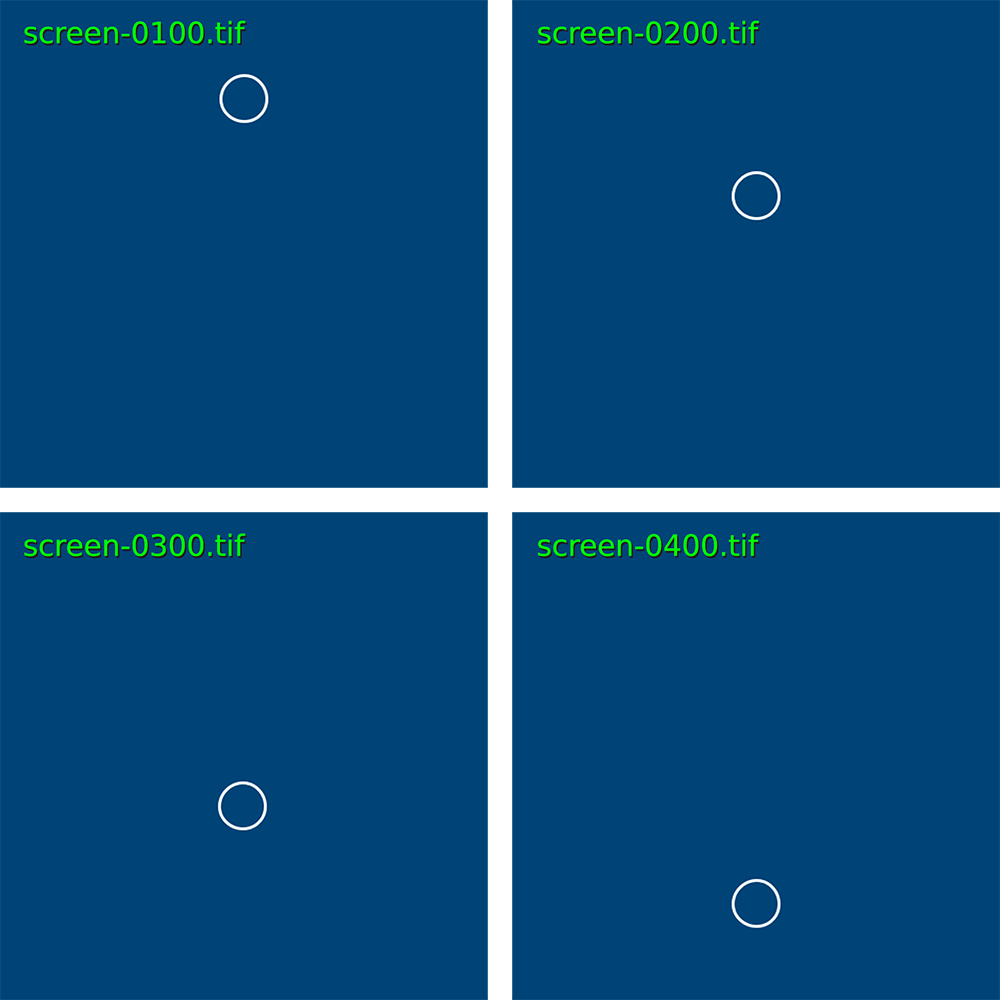Arranged left-to-right, top-to-bottom: frames 100, 200, 300 and 400.

If you wish to save the file using a different name, and/or in some format other than TIFF – such as JPEG, PNG, or TARGA – refer to the saveFrame() reference entry.

DVD players commonly feature a bouncing DVD logo as a screensaver, appearing after a given period of inactivity. You may also have seen some variation of this on another digital device, albeit with a different graphic. Intriguingly, people often find themselves staring at the pointless animation in the hope of witnessing the logo land perfectly in the corner of the screen. If you’re interested to know how long you can expect to wait for such an occurrence, refer to this excellent Lost Math Lessons article.

Create a new sketch and save it as “dvd_screensaver”. Within this, create a “data” folder. Download this “dvd-logo.png” file and place it in the data folder:

dvd-logo.png

Add the following code:

The only the unfamiliar line is the logo = None. This line defines the logo variable as an empty container in the global scope, which is then assigned a graphic in setup() function. Run the sketch.The motion trail indicates the left-to-right movement of the DVD logo. DVD Forum [Public domain / filled in blue], from Wikimedia Commons

The logo should begin moving at an angle, rebounding off every wall it encounters. Your challenge is to complete the task.

Perhaps consider randomising the starting angle.

Transformations

Processing’s transform functions provide a convenient means of dealing with complex geometric transformations. Such operations include scaling, rotation, shearing, and translation. Suppose that you wish to rotate and scale the star shape depicted at the top-left, such that it results in the bottom-right:

The code for the untransformed star’s ten vertices look something like this:

To calculate the coordinates for the rotated + scaled vertex positions, a matrix is required.

Matrices

In mathematics, a matrix (plural: matrices) is a rectangular array of values. As an example, here is a two-by-three matrix (2 rows, 3 columns):

$\left[\begin{array}{ccc}2& 5& 12\\ 19& 9& 7\end{array}\right]$

Given that digital images are essentially rectangular grids of pixels, it is easy to imagine how matrices are used extensively in graphics processing. That said, matrices are applied in various fields of mathematics and other sciences.

To step you through how matrices operate, we’ll take a look at a few practical examples. Things get pretty mathematical here, but the idea is to grasp a matrix as a concept. Following this section, you will be introduced to Processing’s built-in matrix functions – meaning you will no longer need to perform the calculations yourself.

Create a new sketch and save it as “matrices”. Within the sketch’s folder, create a “data” subfolder containing a copy of the grid.png and grid-overlay.png files:

Add the following code:

In Python, semicolons (;) serve as a substitute for new lines. The x/y/w/h variable assignments have been arranged in the above manner as a matter of style. If you wish to avoid the semicolons, you may write each variable on its own line. The x/y values represent the coordinates for the top-left corner of the square; the w/h variables, its width and height. Run the sketch and confirm that your display window matches the image below.

Matrices operate on vertices, whereas the rect() function does not. To move forward, substitute the rect() function with a quad().

The output appears the same but is now based on vertices. Of course, this code does not make use of Processing’s vertex() function, but each x-y pair of values comprises a vertex nonetheless.

Translate

The first transformation you will perform is a translation; this involves moving the square a given distance in some direction. It’s an easy enough task to accomplish without a matrix, but also ideal for introducing how matrices work.

Firstly, take note of how the top-left vertex (x, y) determines the positions of the other three vertices. Therefore the only matrix operation you need to perform is on the top-left vertex – the remaining vertices can be calculated by adding the relevant w and h values. The matrix you are manipulating can, hence, be expressed as:

$\left[\begin{array}{c}x\\ y\end{array}\right]$

Or, if you substitute the variable values:

$\left[\begin{array}{c}400\\ 200\end{array}\right]$

To translate a matrix, add (or subtract) another matrix. To perform a matrix addition, add-up the values of each row:

$\left[\begin{array}{c}x\\ y\end{array}\right]+\left[\begin{array}{c}a\\ b\end{array}\right]=\left[\begin{array}{c}x+a\\ y+b\end{array}\right]$

Append the following code – namely: two new variables representing values a and b (the distance the shape must move horizontally and vertically); a yellow stroke; and a new quad() that integrates the matrix calculations within its arguments:

Expressed in matrix notation, this is:

$\left[\begin{array}{c}400\\ 200\end{array}\right]+\left[\begin{array}{c}100\\ -80\end{array}\right]=\left[\begin{array}{c}400+100\\ 200+-80\end{array}\right]$

Run the sketch. The new yellow square is drawn 100 pixels further right and 80 pixels closer to the top edge than the original.

Scale

To scale a shape, one must multiply the matrix you intend to transform by one describing a transformation. In mathematical notation, this can be expressed as:

$\left[\begin{array}{c}x\\ y\end{array}\right]×\left[\begin{array}{cc}a& b\\ c& d\end{array}\right]$

And this is the point where the power of matrices becomes evident! Depending on the values you substitute for a, b, c, and d, the result will be either a scale, reflect, squeeze, rotate, or shear operation. Take a moment to study how matrix multiplication is performed:

$\left[\begin{array}{c}x\\ y\end{array}\right]×\left[\begin{array}{cc}a& b\\ c& d\end{array}\right]=\left[\begin{array}{c}\left(x·a\right)+\left(y·b\right)\\ \left(x·c\right)+\left(y·d\right)\end{array}\right]$

To perform a scale, value a multiplies the width, and value d multiplies the height. To half the square’s width and height, use a matrix where both a and d are equal to 0.5:

$\left[\begin{array}{c}400\\ 200\end{array}\right]×\left[\begin{array}{cc}0.5& 0\\ 0& 0.5\end{array}\right]=\left[\begin{array}{c}\left(400×0.5\right)+\left(\mathrm{200}×0\right)\\ \left(400×0\right)+\left(\mathrm{200}×0.5\right)\end{array}\right]$

Add some code that draws a new orange square half the size of the white one:

Run the sketch. The orange square is half the size of the white square, but the position has also changed:

To reveal why the position changes, add the grid-overlay.png file to your code – but halve its size using a third and fourth image() argument:

As evidenced by the grid overlay, when one performs a scale transformation, the entire grid upon which the shape is plotted scales toward the origin (0,0).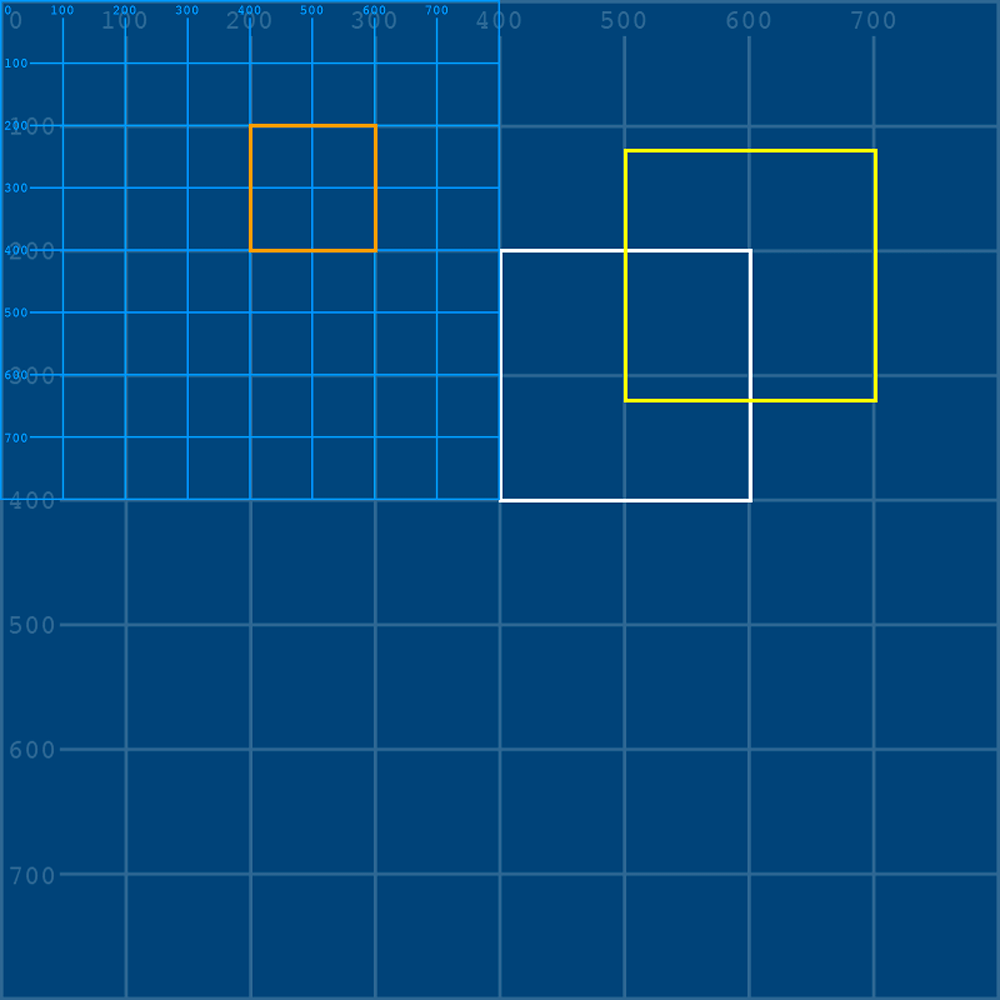The brighter blue lines and numbers are those of the grid-overlay.png file.

Scaling need not be proportionate. Comment out the grid-overlay image, and adjust your a and b variables for a distorted square:

Expressed in matrix notation, this is:

$\left[\begin{array}{c}400\\ 200\end{array}\right]×\left[\begin{array}{cc}0.3& 0\\ 0& 1.8\end{array}\right]=\left[\begin{array}{c}\left(400×0.3\right)+\left(\mathrm{200}×0\right)\\ \left(400×0\right)+\left(\mathrm{200}×1.8\right)\end{array}\right]$

Reflect

Reflecting a shape is a matter of scaling one axis by a negative value; then multiplying the other by 1.

For a horizontal reflection use:

$\left[\begin{array}{c}x\\ y\end{array}\right]×\left[\begin{array}{cc}-a& 0\\ 0& 1\end{array}\right]$

And for a vertical reflection:

$\left[\begin{array}{c}x\\ y\end{array}\right]×\left[\begin{array}{cc}1& 0\\ 0& -d\end{array}\right]$

Be aware, though: reflection operations are relative to the origin (0,0). As an example, add a new red square that is a horizontal reflection of the white original:

Expressed in matrix notation, this is:

$\left[\begin{array}{c}400\\ 200\end{array}\right]×\left[\begin{array}{cc}-1& 0\\ 0& 1\end{array}\right]=\left[\begin{array}{c}\left(400×-1\right)+\left(\mathrm{200}×0\right)\\ \left(400×0\right)+\left(\mathrm{200}×1\right)\end{array}\right]$

The result is a square with a width of -200, drawn from a starting coordinate of (-400,200). As this lies beyond left-edge of the display window, it cannot be seen.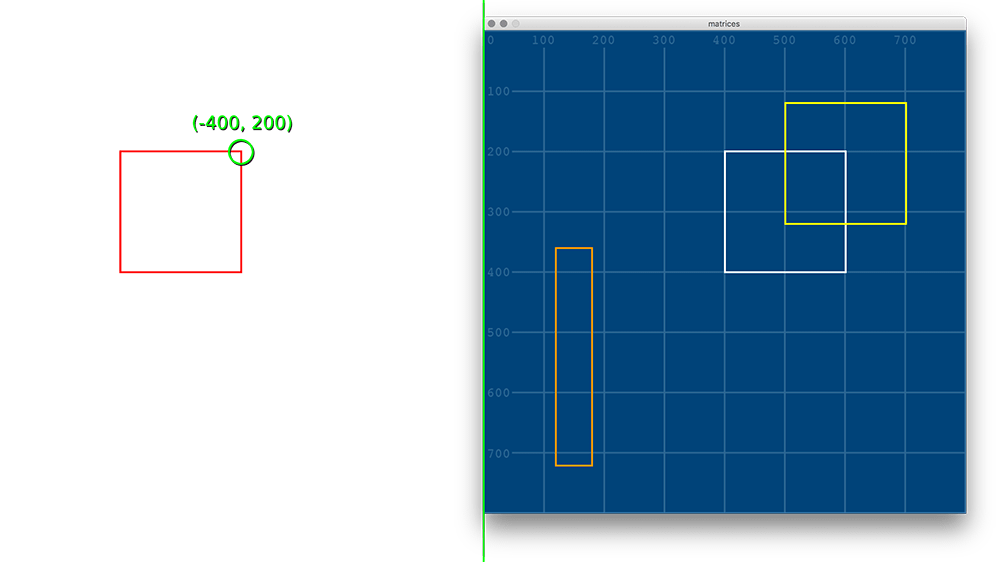The green line indicates the axis of horizontal reflection.

Rotate

Rotation transformations require the trigonometric functions cos() and sin(). Recall, though, that Processing deals in radians rather than degrees, so any arguments you pass these functions must be expressed in radians.

Add a pink version of the white square, rotated 45 degrees (roughly 0.785 radians).

Expressed in matrix notation, this is:

$\left[\begin{array}{c}400\\ 200\end{array}\right]×\left[\begin{array}{cc}cos\left(0.785\right)& -sin\left(0.785\right)\\ sin\left(0.785\right)& cos\left(0.785\right)\end{array}\right]$

The result is a ‘top-left’ coordinate of roughly (141, 424).

$\left[\begin{array}{c}282.96+-141.37\\ 282.73+141.47\end{array}\right]=\left[\begin{array}{c}141.58\\ 424.2\end{array}\right]$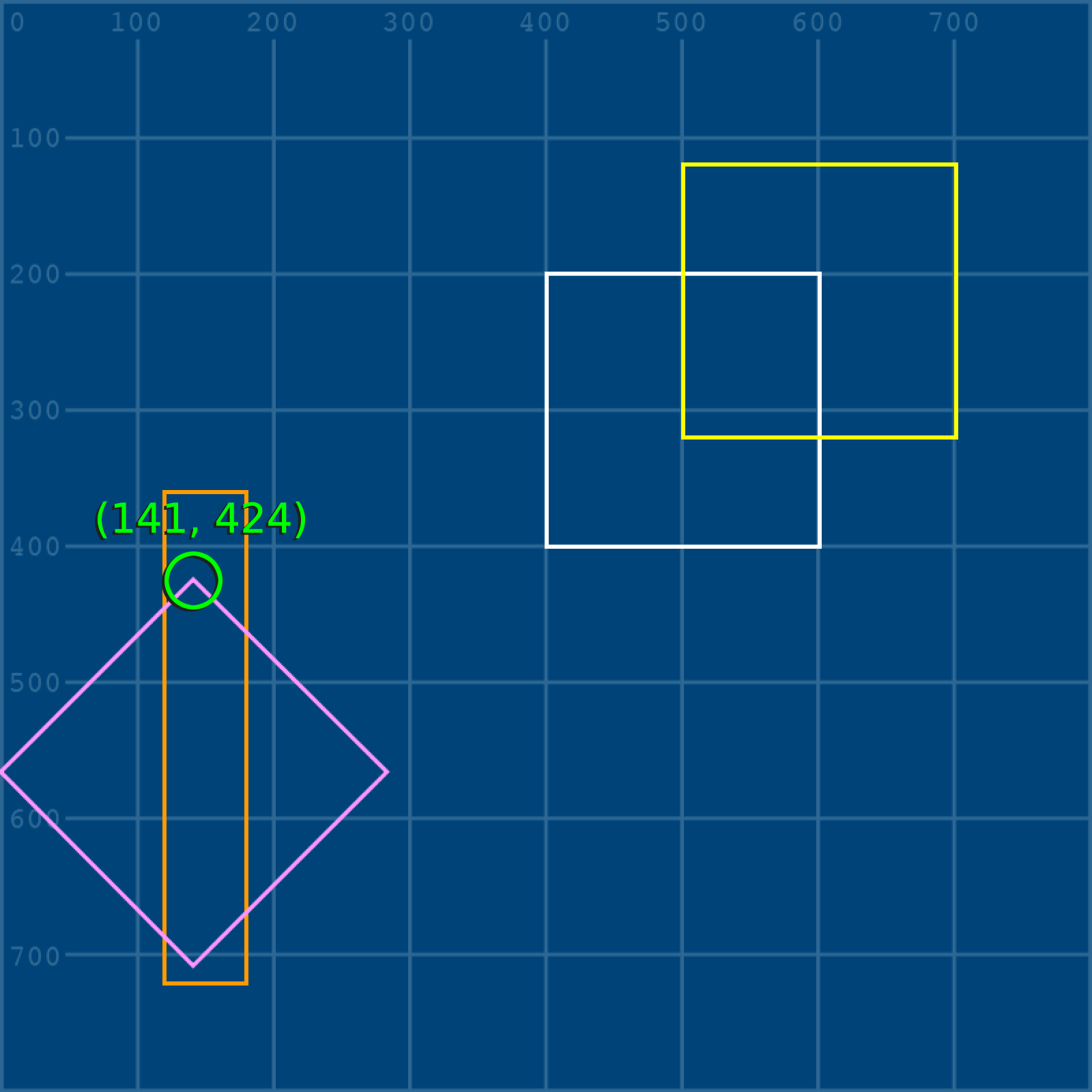With the square rotated 45 degrees, the top-left corner appears in a top-centre position.

As with the other transformations, the axis runs through (0,0). To better visualise this, here is the rotation represented with a grid-overlay graphic:

Shear

Shearing a shape slants/skews it along the horizontal or vertical axis. The area (or volume), however, remains constant.

To shear horizontally, use:

$\left[\begin{array}{c}x\\ y\end{array}\right]×\left[\begin{array}{cc}1& b\\ 0& 1\end{array}\right]$

And for a vertical shear:

$\left[\begin{array}{c}x\\ y\end{array}\right]×\left[\begin{array}{cc}1& 0\\ c& 1\end{array}\right]$

Add a red version of the white square, sheared vertically using a coefficient of 0.4.

Expressed in matrix notation, this is:

$\left[\begin{array}{c}400\\ 200\end{array}\right]×\left[\begin{array}{cc}1& 0\\ 0.4& 1\end{array}\right]=\left[\begin{array}{c}\left(400×1\right)+\left(\mathrm{200}×0\right)\\ \left(400×0.4\right)+\left(\mathrm{200}×1\right)\end{array}\right]$

Below is the result, along with a grid-overlay and x-y coordinate label:

This section has introduced some fundamental transformation concepts using matrices while avoiding anything larger than 2 × 2 matrix. If you want to handle larger matrices, take a look at Python’s NumPy library.

In programming, a library is a collection of prewritten code. Instead of you having to write everything from scratch, Python provides a system for utilising packages of other people’s code. We will be leveraging a few different libraries in these lessons.

Next up: performing all of the above transformations without having to worry about the math!

Processing Transform Functions

After having done transformations the hard way, grasping Processing’s transform functions is a breeze.

Create a new sketch and save it as “transform_functions”. Within the sketch’s folder, create a “data” subfolder containing a copy of the grid.png and grid-overlay.png files:

Add the following setup code (note that grid-overlay.png is loaded but not drawn):

Add a white rectangle, drawn in the same position as that of the last sketch. Because you will be using Processing’s built-in transform functions, a rect() will work fine, i.e. there is no need to use a quad() unless you prefer to.

Add a translate(100,-80) function and a duplicate rect() in yellow:

Add a square, 100 × 100 pixels in width/height, with an x-y coordinate of zero:

Run the sketch. The red square can be seen cut-off near the top-left of the display window. The shapes do not change position – rather it is the coordinate system that does. To better visualise this behaviour, draw the grid-overlay.png after the translate() function:

To return the red square to the top-left corner, one could add a translate(-100,80) to offset the prior one – or better yet, isolate the translation. Wrap the translate, image, and yellow square code in a pushMatrix() and popMatrix() function. These will create a new matrix stack within which only the nested shapes (a yellow square, for now) are translated:

This makes for a useful drawing approach – moving the coordinate system to avoid keeping track using variables, then adding a popMatrix() to return to the original coordinate system. It ultimately depends on what works best for what you are drawing.

In addition to translate(), Processing’s 2D transform functions include, rotate(), scale(), shearX(), and shearY().

What is more, you may combine these as you wish. Add further transform functions to the existing matrix stack:

You will be using a mix of coordinate tracking and transformation techniques in the tasks to come.

Time and Date

There are twenty-four hours in a day. But why is this not ten, twenty, a hundred, or some other more ‘rounded’ number? To make things more confusing, these twenty-four hours are then split into twelve AM and twelve PM hours. To understand why this is, one must look back to where the system first originated – around Mesopotamia and Ancient Egypt, circa 1500 BC. These civilisations relied on sundials and water-clocks for day- and night-time timekeeping, which explains the need for the two (AM/PM) cycles. The twelve figure arises from an ancient finger-counting system, where one uses the thumb to count up to twelve on the three bones of each finger.

Not only does this explain the origins of the 12-hour clock, but also the dozen grouping system. Twelve also happens to have the most divisors of any number below eighteen.

If the twelve-hour clocks make time tricky to deal with, time zones only complicate matters further. Given that the earth is a spinning ball circling the sun, it’s always noon along some longitudinal arc of the planet’s surface. Because the earth must rotate a full 360 degrees to complete one day, ‘noon’ moves across the planet’s surface at 15 degrees (360 ÷ 24) each hour, giving rise to 24 solar time zones. But whether or not your watch reads 12:00 depends on the local time zone in which you find yourself. For example, Australia has three such local time zones whereas China has one, despite the latter straddling four solar time zones.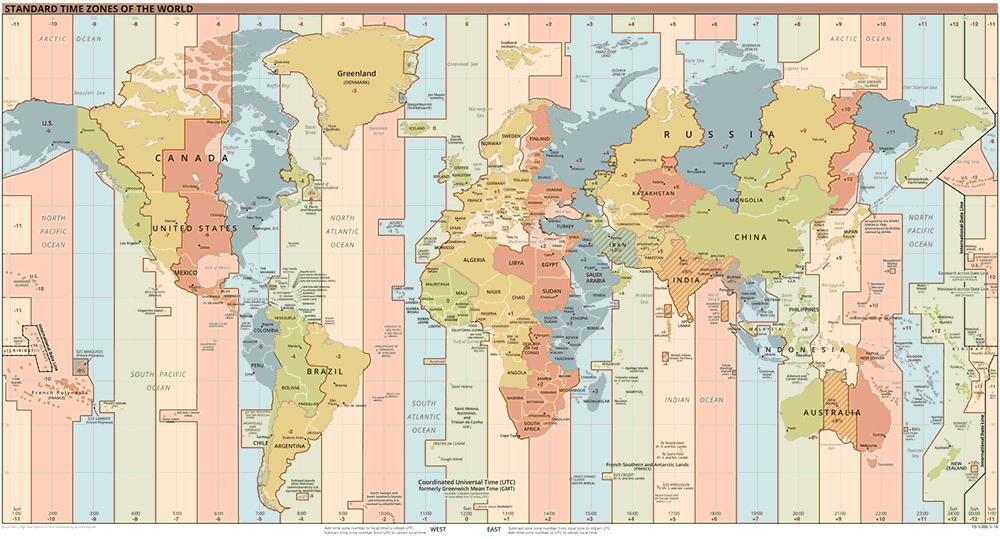Standard world time zones. TimeZonesBoy [Public domain], via Wikimedia Commons

Then there are day-light savings hours to contend with (where one day in each year must be 23 hours long; and another, 25). Never mind that each year is comprised of 52 weeks, 12 months (of varying lengths), 365 days … oh, and what about leap years? All of these factors make handling time a complicated task – but, fortunately, Processing provides a number of functions that perform the tricky calculations for you.

Firstly, though, an introduction to UTC (Coordinated Universal Time) will help make time zones more manageable. UTC – which is interchangeable with GMT – lies on zero degrees longitude and is never adjusted for daylight savings. Local time zones can be expressed using a UTC offset, for example:

 China: UTC+08:00 India: UTC+05:30 Uruguay: UTC-03:00

The Python datetime library provides a utcnow() method for retrieving UTC timestamps:

Unix timestamps represent the number of seconds that have elapsed since 00:00:00, January 1st, 1970 (UTC):

The datetime and time libraries include a complete set of functions for manipulating and managing time data, and depending on what you wish to accomplish you may elect one toolset over the other. However, Processing’s built-in functions that may prove easier to use.

Processing Date and Time Functions

To begin exploring Processing time and date functions, create a new sketch and save it as “date_and_time”. Add the following code:

The date and time functions above communicate with your computer clock (local time). Each function returns an integer value, as your Console output will confirm. The string interpolation approach cuts-out the need for multiple + operators, replacing each pair of {} braces with its respective .format() argument.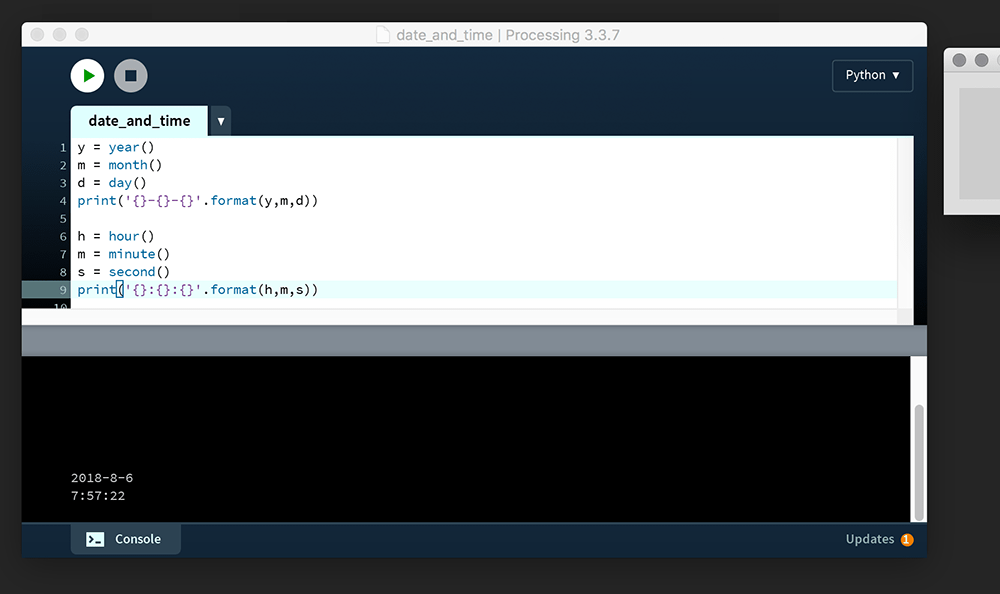The figures in your Console will reflect the point in time at which you run your sketch. In this instance, the Console reads: 2018-8-6 7:57:22

Unlike the functions above, millis() does not fetch date and time values from your clock – but instead returns the number of milliseconds (thousands of a second) that have elapsed since starting the program. Add the following code:

Run this code. The ms value is unlikely to exceed 700. However, the number of milliseconds printed to Console will vary depending on any code executed prior to the millis() function call. For example, add a for loop and have it draw a million squares:

The ms value is now likely to exceed 1000. I say “likely”, because your computer specs and any processes running in the background will have an influence. As a case in point, running this code on my laptop while transcoding a video file added around another thousand milliseconds to the readout.

The millis() function operates independently of frame rate (which is prone to fluctuation). To monitor how many frames have elapsed, use the frameCount system variable.

Sprite Sheet Animation

A sprite is a single graphic comprised of pixels. Multiple sprites can be combined to create a scene like that of this Mario Bros. level:

Study the screenshot above; notice how the blue bricks are all clones of one another. Rather than place a unified environment image into the scene, the developer repeats a single blue brick at the positions necessary to form the platforms. The paved red surface is also formed using a repeating tile. Mario, the turtle, and the fireball are also sprites, although, unlike the environmental sprites, these are animated. Every frame that comprises Mario’s walk-cycle is packed into a single sprite sheet – like in this Nyan Cat example:

By packing every frame into a single image, the developer need only load a single sprite sheet graphic, thus reducing memory and processing overhead. Additionally, all of the background elements are packed into a separate sprite sheet of their own. The technique dates back to early video game development but has since been utilised in other domains, such as web development.

To create a sprite sheet animation of your own, create a new sketch and save it as “spritesheet_animation”. Within this, create a “data” folder. Download a copy of the Nyan Cat sprite sheet and place it in the data folder:

nyancat-spritesheet.gif

Add the following code:

A sprite sheet must move about within a containing box, as if behind a sort of mask. In this instance, the display window will serve as that ‘mask’. The nyancat-spritesheet.gif is 1500 pixels wide and 138 pixels high. This means that each frame is 300 pixels wide (1500 ÷ 5), hence the size(300,138) line. To advance a frame, the nyancat-spritesheet.gif graphic needs to be shifted 300 pixels further left with each iteration of draw(). The position can be controlled using frameCount, but combined with a modulo operation to bring it back to zero every fifth frame:

Run the sketch to verify that Nyan Cat loops seamlessly.

This was an elementary introduction to sprite sheets. It’s hardly an ideal approach (that modulo operation only grows more demanding as the frameCount increases). Most sprite sheets are grid-like in appearance, with graphics arranged in rows and columns. Should you wish to create 2D game – using Processing or another platform – you will want to research this topic further.

Animated Trigonometry

It is assumed that you possess some (if only a very little bit of) trigonometry knowledge. What follows is a practical and visual introduction to trigonometry in motion. Clock, metronome, and piston mechanisms all exhibit elegant rhythms that can be reproduced using sine and cosine functions.

Trigonometry focuses on triangles but is also associated with circles. A refresher on radians is required before venturing further, and what better way to link angles and circles than analogue clocks?

Creating a digital clock in Processing is a simple matter of combining time and text functions. For an analogue clock, however, one must convert the hours, minutes, and seconds into angles of rotation.

Create a new sketch and save it as “analogue_clock”. To help you along, begin with some code that prints out Processing’s pi constants:

These will prove handy for programming your clock. For instance, rather than entering PI/2 each time you want to rotate something 90 degrees, you can instead use HALF_PI. Regarding TAU … well, there is this big, nerdy mathematician war raging over whether it is better to use pi or tau. Basically, π represents only half a circle in radians, so 2π tends to spring up in formulae all over the place, i.e. there are 2π radians in a circle. In 2001 it was proposed that a new constant be devised to represent a full circle; in 2010 it was decided that this value would be represented using the tau symbol (τ). The table below represents equivalent expressions using TAU and PI constants, additionally listing their approximate decimal values:

 TAU = TWO_PI = 6.284 TAU/2 = PI = 3.142 TAU/4 = HALF_PI = 1.571 TAU/8 = QUARTER_PI = 0.785

To begin the clock, draw the face and hour hand:

The hour hand currently rests along zero radians (pointing East). Recall that when drawing arcs, the angle opens clockwise (southward). However, our clock will be offset by three hours should the hand begin from a 3 o’clock position. Correct this using a rotate() function:

The next step is to calculate how many radians the hand advances with each hour. Consider that a complete rotation is tau radians; therefore one hour equals tau over __? Multiply this by the hour() function and use a push/popMatrix to perform the rotation. Once the hour hand is functional, add the minute and second hands. Your finished clock will look something like this:

With a good grasp of radians, we can move onto trigonometric functions. Should you ever need to convert between radians and degrees, look up the radians() and degrees() functions.

SOH-CAH-TOA

Remember SOHCAHTOA (sounded out phonetically as sock-uh-toe-uh)? That mnemonic device to help you remember the sine, cosine, and tangent ratios? Remember how you thought you’d never use it again after leaving school? To refresh your memory, the acronym stands for:

 sine = opposite ÷ hypotenuse cosine = adjacent ÷ hypotenuse tangent = opposite ÷ adjacentA simple trigonometric triangle. Note the naming of each side relative to angle A. TheOtherJesse [Public domain], from Wikimedia Commons

Remember unit circles? A unit circle is a circle with a radius of one:

In the above illustration, angle theta (θ) is equal to 45 degrees – or roughly 0.785 radians – or to be more accurate, pi over 4, which may be expressed as QUARTER_PI in Processing. We will return to the unit circle in a moment. For now, create a new sketch and save it as “sohcahtoa”. Add the following code:

A unit circle would be far too small to work with, hence the inclusion of an s variable for scale. Rather than dealing with a radius of 1, we now have a circle with a radius of 200. Provided all coordinates are scaled by the same factor, the trigonometry calculations will work fine. Run the code. The Console will display lines of (0.0, 1.0) pairs. To avoid printing lengthy floating-point values, these have been rounded to one decimal place. Within the line() function’s arguments, x and y are scaled by s – an integer of 200 – to calculate the line’s endpoint:

Increase the angle slightly, then run the code to see what happens:

The angle opens in a clockwise direction. To reverse this, multiply the y-coordinate by negative one. To further mimic the unit circle illustration, set the theta variable to 45 degrees (using radians, of course).

From the Console output, one can see that sin θ returns a value of 0.7, as does cos θ. Therefore, where theta equals QUARTER_PI, sin and cos are equal – but these values drift apart as you increase/decrease the angle. The concept can be illustrated using a unit circle:Top-left: θ=0; top-right: θ=π÷2; bottom-left: θ=π÷4; bottom-right: θ=π+0.3. These pairs can be multiplied to draw a circle of any radius

To understand how this all works, click the image below and watch the cool animation for about ten seconds.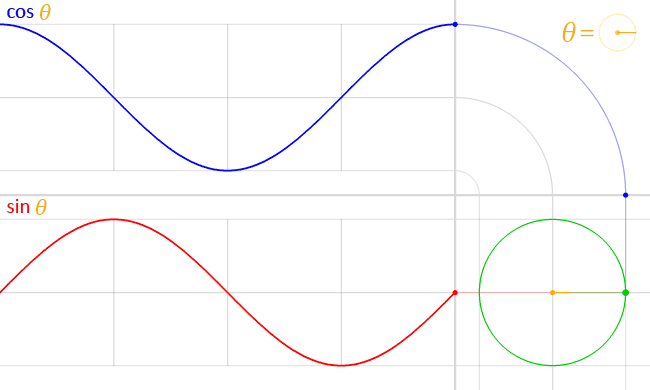Click the image to start the animation. LucasVB [Public domain], from Wikimedia Commons

The frame below captures the moment where θ reaches 45 degrees (QUARTER_PI radians).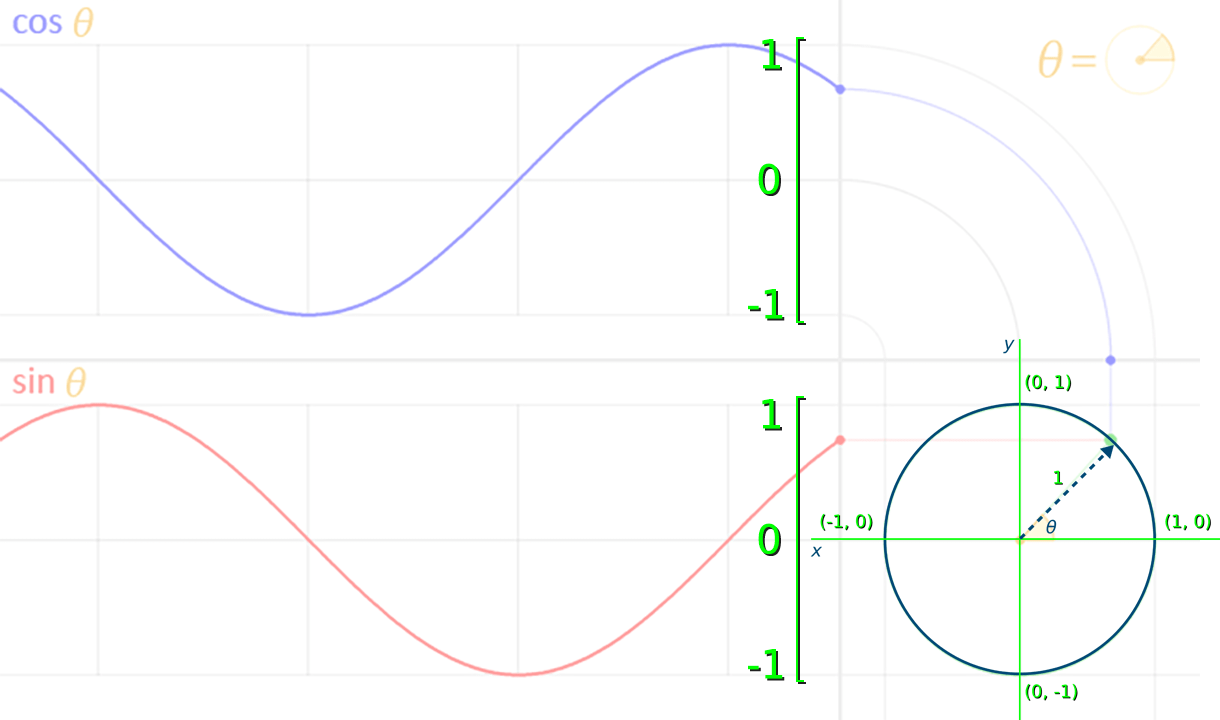The unit circle illustration has been placed over the animated version.

The value for sin θ – the red dot at the end of the red wave – is equal to roughly 0.7. Take note of how the sine function returns values between 1 and -1 that indicate the y-coordinate of where the arrow touches the circle’s circumference. The blue cos wave also oscillates 1 and -1, representing the arrow tip’s x-coordinate.

Increment theta by 0.05 with each new frame, and a smaller circle to capture the sine wave’s vertical motion: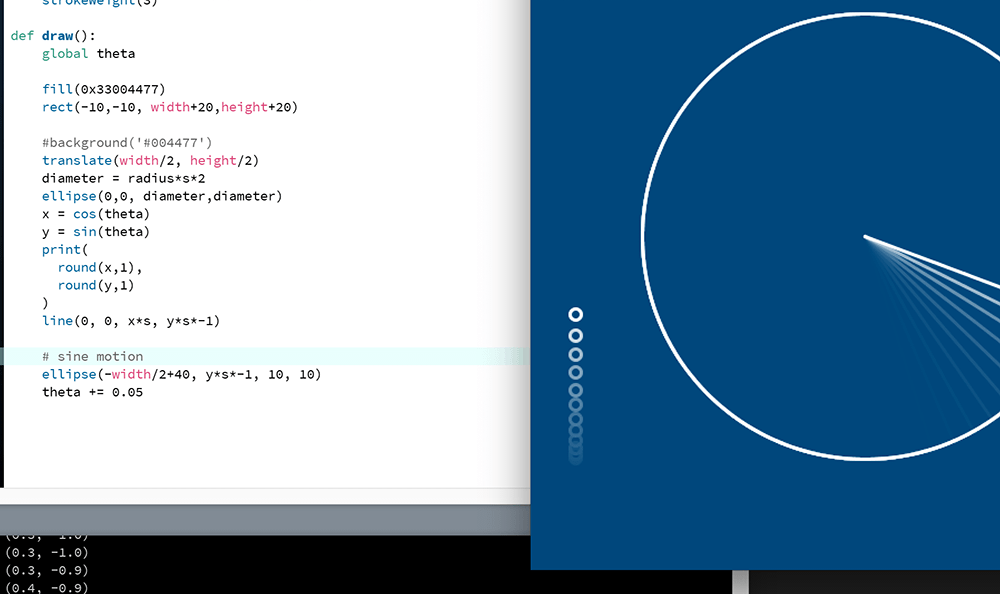The small circle moves up-and-down periodically, moving fastest as it crosses the circle's centre.

Add another small circle to capture the cosine wave’s horizontal motion:

And, finally, add another small circle at the point where the radius connects to the circumference:

Sine (and cosine) wave patterns are studied in various fields, including physics, engineering, and signal processing.

What about the …-TOA part?

Tangent (tan) functions do not produce wave-like graphs. Perhaps one of the neatest practical examples of tan is xeyes, albeit an application of tan’s inverse function, arctangent. Since the early days of the Linux X Window System, developers have included a feature that places a pair of eyes somewhere on the desktop to help users locate the mouse cursor. This is especially handy for multi-head arrangements where there is some distance between displays.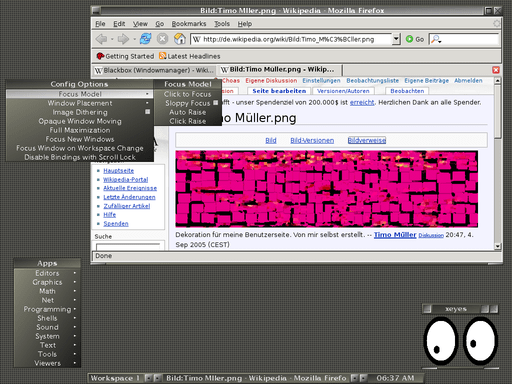The (x)eyes, at the lower-right, rotate to follow the mouse cursor. The original uploader was Choas at German Wikipedia. [GPL], via Wikimedia Commons

Processing provides a 2-argument arctangent function called atan2() which can be used to find an angle between two points. To see this in action, create a pair of xeyes of your own by adding the following code to your “sohcahtoa” sketch: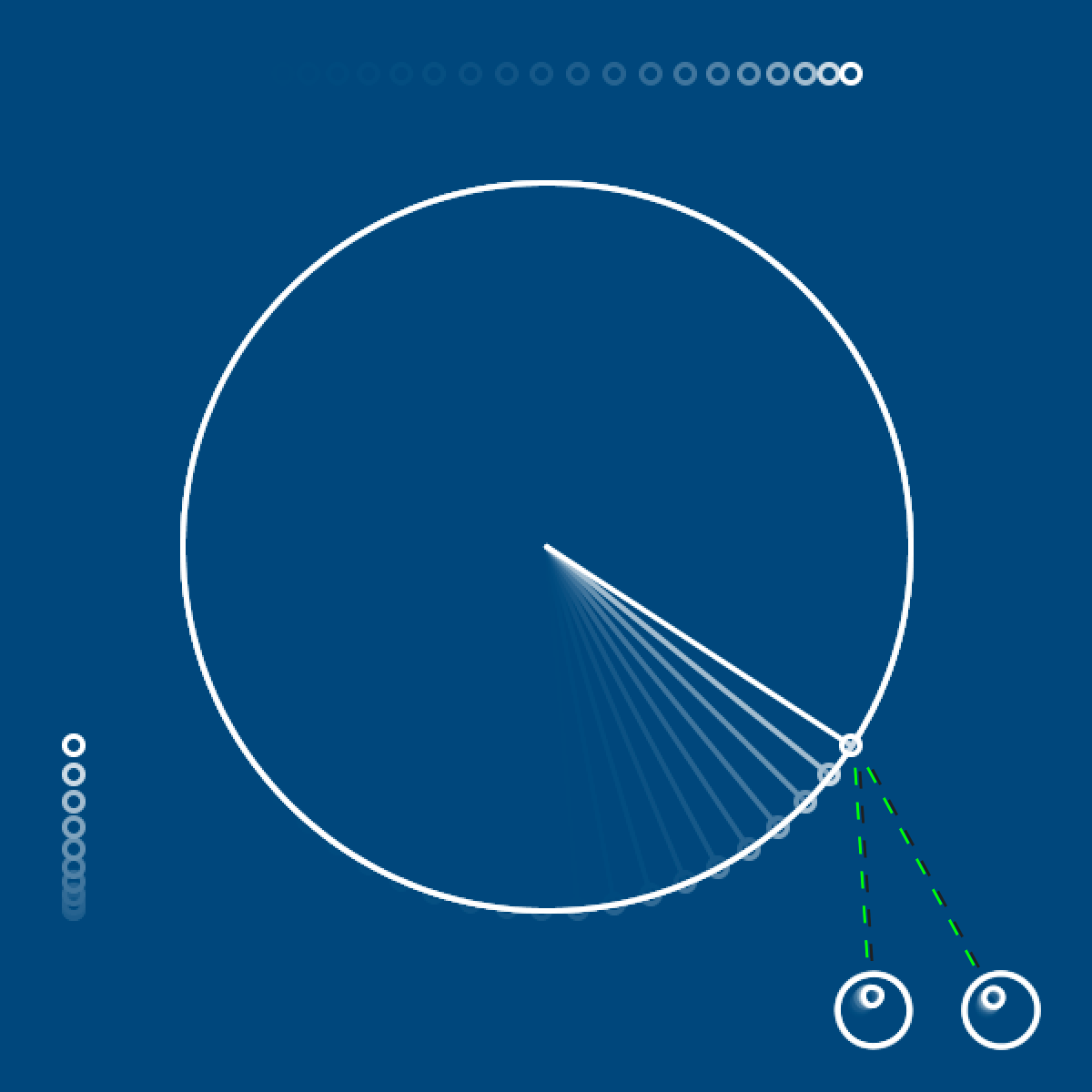The eyes follow the small circle at the end of the line as it races along the boundary.

The distance between the x/y-coordinate pair of the target (the small circle at the end of the radius line) and the origin (an eye) indicate the lengths of the opposite and adjacent sides of a right-angled triangle. The atan2() function accepts these two distance values:

atan2(y-distance, x-distance)

To help make visual sense of this, program a line connecting the target and the right eye:

From here, Processing calculates the ratio between the opposite and adjacent sides. Recall that the tangent is equal to opposite over adjacent (…-TOA). So – whereas the tan function accepts an angle and returns a ratio – it’s inverse, arctangent, accepts the ratio and returns the angle.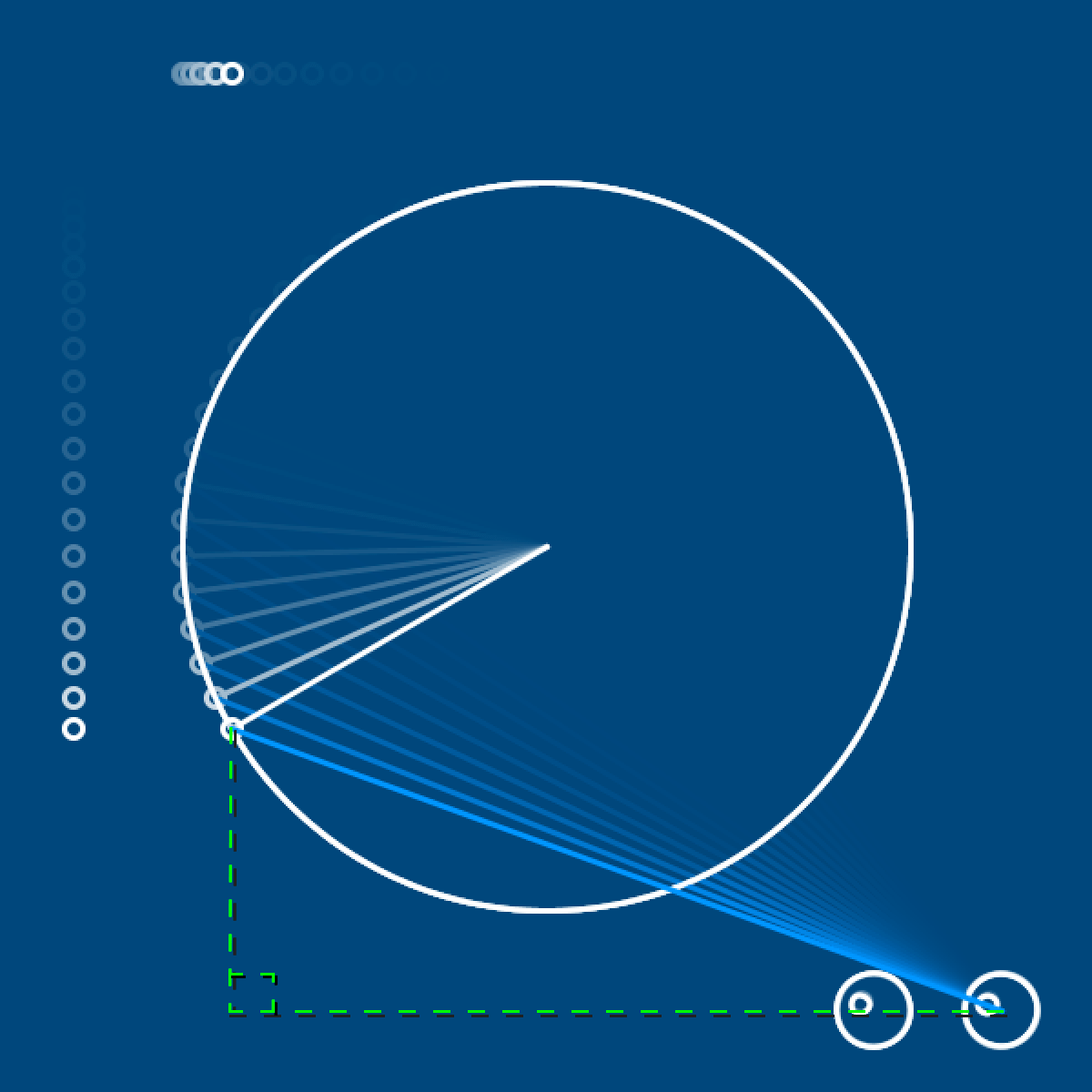The green overlay completes the right-angled triangle formed by pale blue hypotenuse line.

If you have some trouble wrapping your head around atan2(), then do not fret; the upcoming task requires only sin() and cos() functions.

Time for a final challenge before moving onto lesson 05! Recreate the animation below.

Technically speaking, the piston motion in such an engine design is not quite sinusoidal, but very close. If you would like another engine challenge, perhaps try the perfectly sinusoidal Scotch yoke.

Lesson 05

That’s it for lesson 04. If you have made it this far – the next lesson should be a breeze! Lesson 04 was undoubtedly more mathematical than most, and probably longer, too.

You have dealt with string, integer, floating-point, and boolean datatypes. In the next lesson, you will explore datatypes that hold a collection of elements – namely Python list and dictionary types. If you have some programming experience, you may have encountered something similar (arrays) in other languages? If not, do not stress – we will begin with the very basics. As this subject matter works nicely with graphs, we will also explore interesting to visualise data.

Begin Lesson 05: Lists, Dictionaries, and Data

Complete list of Processing.py lessons

• http://lostmathlessons.blogspot.com/2016/03/bouncing-dvd-logo.html
• https://commons.wikimedia.org/wiki/File:DVD_logo.svg
• https://en.wikipedia.org/wiki/Beta_movement